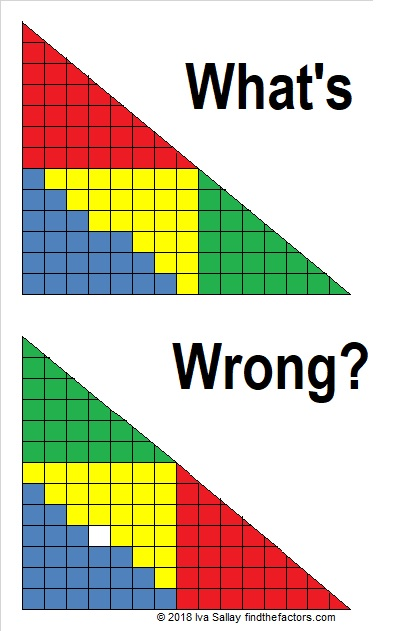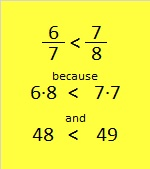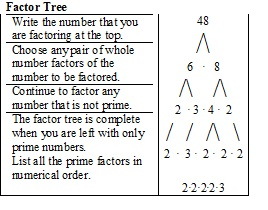1188 How Many Triangles? How Many Factors?

Manually counting ALL the triangles in the graphic below could get confusing. Try counting all the triangles that point up first then all the triangles that point down.Since the number of triangle rows is an even number, you could just use this formula:

n(n+2)(2n+1)/8 = the number of triangles

In this case, n = 16, so the number of triangles is 16×18×33/8 = 1188.

(If the number of rows is an odd number, the formula produces a number with a decimal. In that case, just ignore the decimal and everything after it to get the number of triangles.)

• 1188 is a composite number.
• Prime factorization: 1188 = 2 × 2 × 3 × 3 × 3 × 11, which can be written 1188 = 2² × 3³ × 11
• The exponents in the prime factorization are 2, 3 and 1. Adding one to each and multiplying we get (2 + 1)(3 + 1)(1 + 1) = 3 × 4 × 2 = 24. Therefore 1188 has exactly 24 factors.
• Factors of 1188: 1, 2, 3, 4, 6, 9, 11, 12, 18, 22, 27, 33, 36, 44, 54, 66, 99, 108, 132, 198, 297, 396, 594, 1188
• Factor pairs: 1188 = 1 × 1188, 2 × 594, 3 × 396, 4 × 297, 6 × 198, 9 × 132, 11 × 108, 12 × 99, 18 × 66, 22 × 54, 27 × 44 or 33 × 36
• Taking the factor pair with the largest square number factor, we get √1188 = (√36)(√33) = 6√33 ≈ 34.467381188 has MANY possible factor trees. Here are four of them:1188 is an interesting-looking base 10 number, but it also looks interesting when it is written in some other bases:
It’s 2244 in BASE 8,
990 in BASE 11 because 9(11²) + 9(11) = 9(132) = 1188,
543 in BASE 15,
4A4 in BASE 16 (A is 10 base 10),
XX in BASE 35 (X is 33 base 10) because 33(35) + 33(1) = 33(36) = 1188,
and it’s X0 in BASE 36 because 33(36) = 1188

48 and What’s wrong with this?

Here is a four piece puzzle arranged two different ways. There are 24 blue squares and 24 yellow squares. 24 + 24 = 48. Read on to see why the factors of 48 are significant to this puzzle.Those triangles are some fabulous mathematical slight of hand.  Something is wrong with the puzzle, but what?

Something is fishy with these two triangle puzzles, too:And with these two triangles made with six puzzle pieces!If I had time, I could make an infinite number of these types of puzzles. Why do we get a solid triangle sometimes, but one with a hole in it the other times?

Believe it or not, the answer to the triangle puzzles has something to do with factoring.

Here’s that first puzzle again, but smaller:The first triangle doesn’t have a hole it while the second one does becauseWho would have thought that comparing fractions could turn into a type of magic trick?!!

Both triangles have 48 blue or yellow squares, but the second triangle wants you to think it should have 49.

(Psst….6/7 is also the slope of the green triangle and 7/8 is the slope of the red triangle. Since the two triangles don’t have identical slopes, the big “triangles” formed in the puzzles aren’t really triangles at all.)

I made all the puzzles in this post by comparing fractions. In each case, I simply used two consecutive composite numbers and then factored them and came up with the fractions I wanted to use. The numerators and the denominators of the fractions became the sides of the triangles.

Now, what do you think of this video of a never-ending chocolate bar?

Let me tell you a little bit about the number 48:

48 is a composite number. 48 = 1 x 48, 2 x 24, 3 x 16, 4 x 12, or 6 x 8. Factors of 48: 1, 2, 3, 4, 6, 8, 12, 16, 24, 48. Prime factorization: 48 = 2 x 2 x 2 x 2 x 3, which can also be written 48 = 2⁴ x 3.Sometimes 48 is a clue in the FIND THE FACTORS puzzles. Even though it has many other factors, we use only 6 x 8 for the FIND THE FACTORS 1-10 puzzles and 6 x 8 or 4 x 12 for the FIND THE FACTORS 1-12 puzzles.